Mechanical Engineering - ME 2014 GATE Paper (Practice Test)

# Mechanical Engineering - ME 2014 GATE Paper (Practice Test)

Test Description

## 65 Questions MCQ Test GATE Mechanical (ME) 2023 Mock Test Series | Mechanical Engineering - ME 2014 GATE Paper (Practice Test)

Mechanical Engineering - ME 2014 GATE Paper (Practice Test) for Mechanical Engineering 2023 is part of GATE Mechanical (ME) 2023 Mock Test Series preparation. The Mechanical Engineering - ME 2014 GATE Paper (Practice Test) questions and answers have been prepared according to the Mechanical Engineering exam syllabus.The Mechanical Engineering - ME 2014 GATE Paper (Practice Test) MCQs are made for Mechanical Engineering 2023 Exam. Find important definitions, questions, notes, meanings, examples, exercises, MCQs and online tests for Mechanical Engineering - ME 2014 GATE Paper (Practice Test) below.
Solutions of Mechanical Engineering - ME 2014 GATE Paper (Practice Test) questions in English are available as part of our GATE Mechanical (ME) 2023 Mock Test Series for Mechanical Engineering & Mechanical Engineering - ME 2014 GATE Paper (Practice Test) solutions in Hindi for GATE Mechanical (ME) 2023 Mock Test Series course. Download more important topics, notes, lectures and mock test series for Mechanical Engineering Exam by signing up for free. Attempt Mechanical Engineering - ME 2014 GATE Paper (Practice Test) | 65 questions in 180 minutes | Mock test for Mechanical Engineering preparation | Free important questions MCQ to study GATE Mechanical (ME) 2023 Mock Test Series for Mechanical Engineering Exam | Download free PDF with solutions
 1 Crore+ students have signed up on EduRev. Have you?
Mechanical Engineering - ME 2014 GATE Paper (Practice Test) - Question 1

### Q. No. 1 – 5 Carry One Mark Each “India is a country of rich heritage and cultural diversity.” Which one of the following facts best supports the claim made in the above sentence?

Detailed Solution for Mechanical Engineering - ME 2014 GATE Paper (Practice Test) - Question 1

Diversity is shown in terms of difference language

Mechanical Engineering - ME 2014 GATE Paper (Practice Test) - Question 2

### The value of one U.S. dollar is 65 Indian Rupees today, compared to 60 last year. The Indian Rupee has ____________.

Mechanical Engineering - ME 2014 GATE Paper (Practice Test) - Question 3

*Answer can only contain numeric values
Mechanical Engineering - ME 2014 GATE Paper (Practice Test) - Question 4

The next term in the series 81, 54, 36, 24 … is ________

(Important - Enter only the numerical value in the answer)

Detailed Solution for Mechanical Engineering - ME 2014 GATE Paper (Practice Test) - Question 4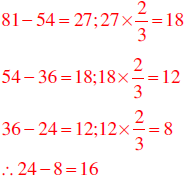Mechanical Engineering - ME 2014 GATE Paper (Practice Test) - Question 5

In which of the following options will the expression P < M be definitely true?

Mechanical Engineering - ME 2014 GATE Paper (Practice Test) - Question 6

Q. No. 6 – 10 Carry Two Marks Each

Q. Find the next term in the sequence: 7G, 11K, 13M, ___

Mechanical Engineering - ME 2014 GATE Paper (Practice Test) - Question 7

The multi-level hierarchical pie chart shows the population of animals in a reserve forest. The correct conclusions from this information are: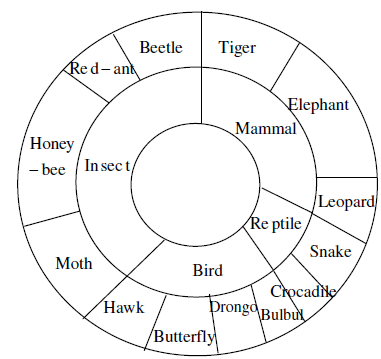(i) Butterflies are birds
(ii) There are more tigers in this forest than red ants
(iii) All reptiles in this forest are either snakes or crocodiles
(iv) Elephants are the largest mammals in this forest

Detailed Solution for Mechanical Engineering - ME 2014 GATE Paper (Practice Test) - Question 7

It is not mentioned that elephant is the largest animal

*Answer can only contain numeric values
Mechanical Engineering - ME 2014 GATE Paper (Practice Test) - Question 8

A man can row at 8 km per hour in still water. If it takes him thrice as long to row upstream, as to row downstream, then find the stream velocity in km per hour.

(Important - Enter only the numerical value in the answer)

Detailed Solution for Mechanical Engineering - ME 2014 GATE Paper (Practice Test) - Question 8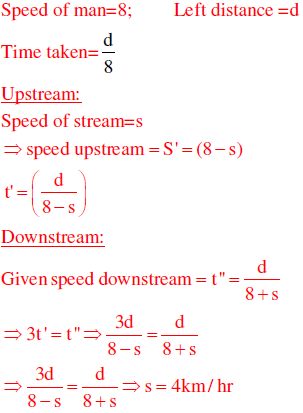*Answer can only contain numeric values
Mechanical Engineering - ME 2014 GATE Paper (Practice Test) - Question 9

A firm producing air purifiers sold 200 units in 2012. The following pie chart presents the share of raw material, labour, energy, plant & machinery, and transportation costs in the total manufacturing cost of the firm in 2012. The expenditure on labour in 2012 is Rs. 4,50,000. In 2013, the raw material expenses increased by 30% and all other expenses increased by 20%. If the company registered a profit of Rs. 10 lakhs in 2012, at what price (in Rs.) was each air purifier sold?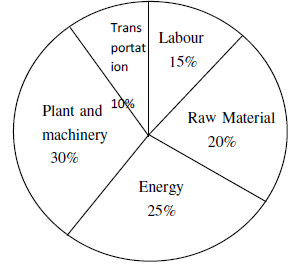(Important - Enter only the numerical value in the answer)

Detailed Solution for Mechanical Engineering - ME 2014 GATE Paper (Practice Test) - Question 9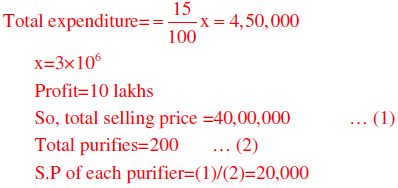*Answer can only contain numeric values
Mechanical Engineering - ME 2014 GATE Paper (Practice Test) - Question 10

A batch of one hundred bulbs is inspected by testing four randomly chosen bulbs. The batch
is rejected if even one of the bulbs is defective. A batch typically has five defective bulbs.
The probability that the current batch is accepted is _________

(Important - Enter only the numerical value in the answer)

Detailed Solution for Mechanical Engineering - ME 2014 GATE Paper (Practice Test) - Question 10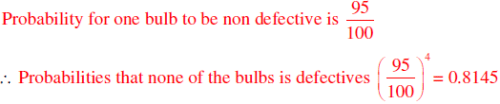Mechanical Engineering - ME 2014 GATE Paper (Practice Test) - Question 11

Q. No. 11 – 35 Carry One Mark Each
1. Consider a 3 × 3 real symmetric matrix S such that two of its eigen values are a = 0 , b = 0 with respective eigen vectors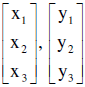. If a = b then x1y1 + x2y2 + x2y2 equals

Detailed Solution for Mechanical Engineering - ME 2014 GATE Paper (Practice Test) - Question 11

We know that the Eigen vectors  corresponding to distinct Eigen values of real symmetric matrix are orthogonal.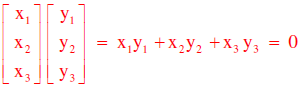Mechanical Engineering - ME 2014 GATE Paper (Practice Test) - Question 12

If a function is continuous at a point,

Detailed Solution for Mechanical Engineering - ME 2014 GATE Paper (Practice Test) - Question 12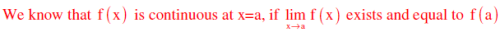Mechanical Engineering - ME 2014 GATE Paper (Practice Test) - Question 13

Divergence of the vector field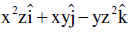at (1, -1, 1) is

Detailed Solution for Mechanical Engineering - ME 2014 GATE Paper (Practice Test) - Question 13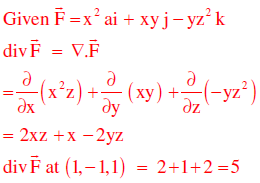*Answer can only contain numeric values
Mechanical Engineering - ME 2014 GATE Paper (Practice Test) - Question 14

A group consists of equal number of men and women. Of this group 20% of the men and 50% of the women are unemployed. If a person is selected at random from this group, the probability of the selected person being employed is _______

(Important - Enter only the numerical value in the answer)

Detailed Solution for Mechanical Engineering - ME 2014 GATE Paper (Practice Test) - Question 14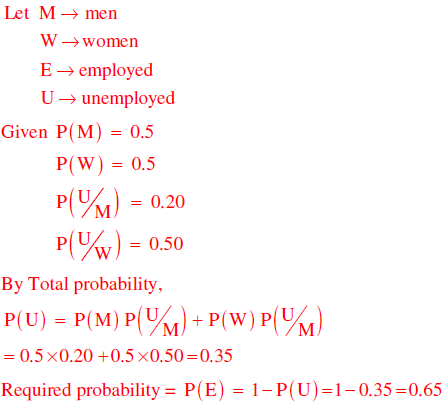*Answer can only contain numeric values
Mechanical Engineering - ME 2014 GATE Paper (Practice Test) - Question 15

The definite integral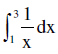is evaluated using Trapezoidal rule with a step size of 1. The correct answer is ________

(Important - Enter only the numerical value in the answer)

Detailed Solution for Mechanical Engineering - ME 2014 GATE Paper (Practice Test) - Question 15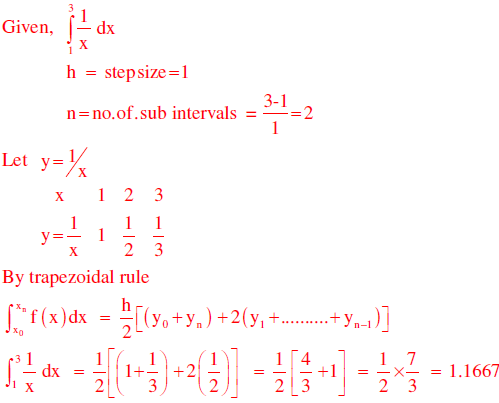*Answer can only contain numeric values
Mechanical Engineering - ME 2014 GATE Paper (Practice Test) - Question 16

A rotating steel shaft is supported at the ends. It is subjected to a point load at the centre. The maximum bending stress developed is 100 MPa. If the yield, ultimate and corrected endurance strength of the shaft material is 300 MPa, 500 MPa and 200 MPa, respectively, then the factor of safety for the shaft is _______

(Important - Enter only the numerical value in the answer)

Detailed Solution for Mechanical Engineering - ME 2014 GATE Paper (Practice Test) - Question 16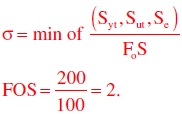*Answer can only contain numeric values
Mechanical Engineering - ME 2014 GATE Paper (Practice Test) - Question 17

Two solid circular shafts of radii R1 and R2 are subjected to same torque. The maximum shear stresses developed in the two shafts are τ1 and τ2 . If R1 / R2=2, then τ2 / τ1 is _______

(Important - Enter only the numerical value in the answer)

Detailed Solution for Mechanical Engineering - ME 2014 GATE Paper (Practice Test) - Question 17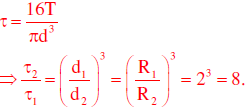Mechanical Engineering - ME 2014 GATE Paper (Practice Test) - Question 18

Consider a single degree-of-freedom system with viscous damping excited by a harmonic force. At resonance, the phase angle (in degree) of the displacement with respect to the exciting force is

Mechanical Engineering - ME 2014 GATE Paper (Practice Test) - Question 19

A mass 1 m of 100 kg travelling with a uniform velocity of 5 m/s along a line collides with a stationary mass m2 of 1000 kg. After the collision, both the masses travel together with the same velocity. The coefficient of restitution is

Detailed Solution for Mechanical Engineering - ME 2014 GATE Paper (Practice Test) - Question 19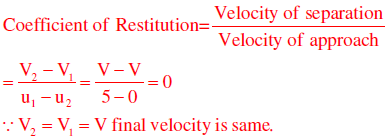Mechanical Engineering - ME 2014 GATE Paper (Practice Test) - Question 20

Which one of following is NOT correct?

Mechanical Engineering - ME 2014 GATE Paper (Practice Test) - Question 21

Gear 2 rotates at 1200 rpm in counter clockwise direction and engages with Gear 3. Gear 3 and Gear 4 are mounted on the same shaft. Gear 5 engages with Gear 4. The numbers of teeth on Gears 2, 3, 4 and 5 are 20, 40, 15 and 30, respectively. The angular speed of Gear 5 is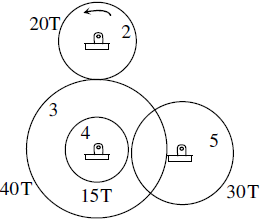Detailed Solution for Mechanical Engineering - ME 2014 GATE Paper (Practice Test) - Question 21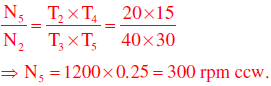Mechanical Engineering - ME 2014 GATE Paper (Practice Test) - Question 22

Consider a long cylindrical tube of inner and outer radii, ri and ro , respectively, length, L and thermal conductivity, k. Its inner and outer surfaces are maintained at Ti and T0, respectively ( Ti > TO ). Assuming one-dimensional steady state heat conduction in the radial direction, the thermal resistance in the wall of the tube is

Detailed Solution for Mechanical Engineering - ME 2014 GATE Paper (Practice Test) - Question 22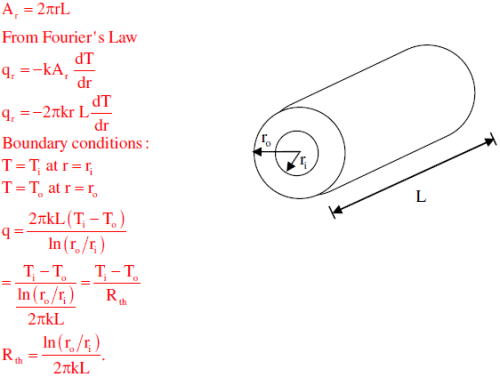Mechanical Engineering - ME 2014 GATE Paper (Practice Test) - Question 23

Which one of the following pairs of equations describes an irreversible heat engine?

Detailed Solution for Mechanical Engineering - ME 2014 GATE Paper (Practice Test) - Question 23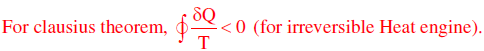Mechanical Engineering - ME 2014 GATE Paper (Practice Test) - Question 24

Consider the turbulent flow of a fluid through a circular pipe of diameter, D. Identify the correct pair of statements.
I. The fluid is well-mixed
II. The fluid is unmixed
III. ReD < 2300
IV. ReD > 2300

Detailed Solution for Mechanical Engineering - ME 2014 GATE Paper (Practice Test) - Question 24

ReD > 2300 means it is a turbulent flow. In turbulent flow, the fluid is well mixed. The fluid is unmixed, for a very-low Reynolds number laminar flow.

Mechanical Engineering - ME 2014 GATE Paper (Practice Test) - Question 25

For a gas turbine power plant, identify the correct pair of statements.

P. Smaller in size compared to steam power plant for same power output

Q. Starts quickly compared to steam power plant

R. Works on the principle of Rankine cycle

S. Good compatibility with solid fuel

Detailed Solution for Mechanical Engineering - ME 2014 GATE Paper (Practice Test) - Question 25

Steam power plants are bulky due to presence of boiler and condenser. Gas turbines are compact, as compressors and turbines are coupled on a common shaft. In steam power plants, boiler takes lot of time to get started, as compared to Gas Turbines.

*Answer can only contain numeric values
Mechanical Engineering - ME 2014 GATE Paper (Practice Test) - Question 26

A source at a temperature of 500 K provides 1000 kJ of heat. The temperature of environment is 27OC. The maximum useful work (in kJ) that can be obtained from the heat source is_______

(Important - Enter only the numerical value in the answer)

Detailed Solution for Mechanical Engineering - ME 2014 GATE Paper (Practice Test) - Question 26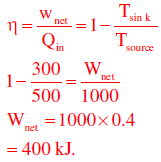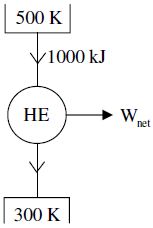*Answer can only contain numeric values
Mechanical Engineering - ME 2014 GATE Paper (Practice Test) - Question 27

A sample of moist air at a total pressure of 85 KPa has a dry bulb temperature of 30°C (saturation vapour pressure of water = 4.24 KPa). If the air sample has a relative humidity of 65%, the absolute humidity (in gram) of water vapour per kg of dry air is _______

(Important - Enter only the numerical value in the answer)

Detailed Solution for Mechanical Engineering - ME 2014 GATE Paper (Practice Test) - Question 27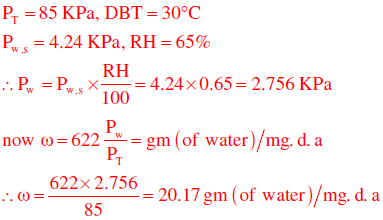Mechanical Engineering - ME 2014 GATE Paper (Practice Test) - Question 28

The process utilizing mainly thermal energy for removing material is

*Answer can only contain numeric values
Mechanical Engineering - ME 2014 GATE Paper (Practice Test) - Question 29

The actual sales of a product in different months of a particular year are given below: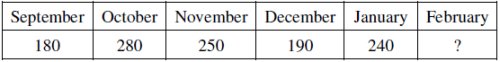The forecast of the sales, using the 4-month moving average method, for the month of February is _______

(Important - Enter only the numerical value in the answer)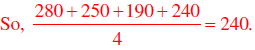Detailed Solution for Mechanical Engineering - ME 2014 GATE Paper (Practice Test) - Question 29

Number of periods = 4, then the past 4 months average sales is fore cast for next 4 months.*Answer can only contain numeric values
Mechanical Engineering - ME 2014 GATE Paper (Practice Test) - Question 30

A straight turning operation is carried out using a single point cutting tool on an AISI 1020 steel rod. The feed is 0.2 mm/rev and the depth of cut is 0.5 mm. The tool has a side cutting edge angle of 60°. The uncut chip thickness (in mm) is _______

(Important - Enter only the numerical value in the answer)

Detailed Solution for Mechanical Engineering - ME 2014 GATE Paper (Practice Test) - Question 30Mechanical Engineering - ME 2014 GATE Paper (Practice Test) - Question 31

A minimal spanning tree in network flow models involves

Detailed Solution for Mechanical Engineering - ME 2014 GATE Paper (Practice Test) - Question 31

A path forms a loop or cycle if it connects a node itself. A spanning tree links all the nodes of network with no loops allowed.

Mechanical Engineering - ME 2014 GATE Paper (Practice Test) - Question 32

A path forms a loop or cycle if it connects a node itself. A spanning tree links all the nodes of network with no loops allowed.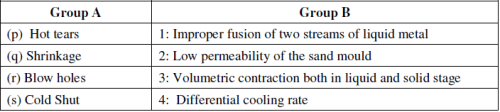Mechanical Engineering - ME 2014 GATE Paper (Practice Test) - Question 33

Cutting tool is much harder than the workpiece. Yet the tool wears out during the tool-work interaction, because

Mechanical Engineering - ME 2014 GATE Paper (Practice Test) - Question 34

The stress-strain curve for mild steel is shown in the figure given below. Choose the correct option referring to both figure and table.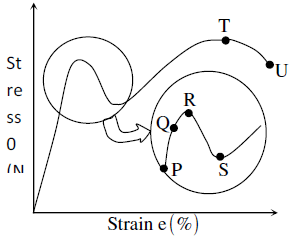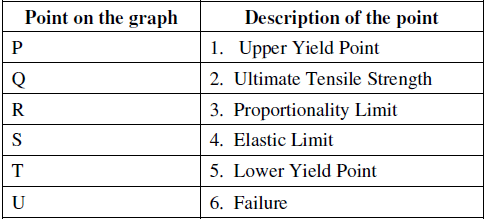Mechanical Engineering - ME 2014 GATE Paper (Practice Test) - Question 35

The hot tearing in a metal casting is due to

Mechanical Engineering - ME 2014 GATE Paper (Practice Test) - Question 36

Q. No. 36 – 65 Carry Two Marks Each

Q. An analytic function of a complex variable z = x + iy is expressed as f(z) = w(x, y) + iv(x, y), where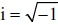. If u(x, y) = x2 – y2, then expression for v(x, y) in terms of x, y and a general constant c would be

Detailed Solution for Mechanical Engineering - ME 2014 GATE Paper (Practice Test) - Question 36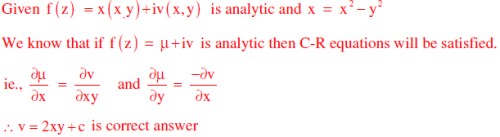Mechanical Engineering - ME 2014 GATE Paper (Practice Test) - Question 37

Consider two solutions x(t) = x1t and x(t) and x(t) = x2(t) of the differential equation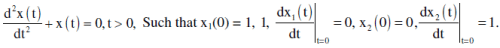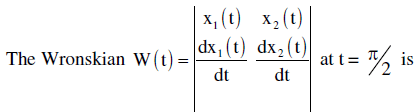Detailed Solution for Mechanical Engineering - ME 2014 GATE Paper (Practice Test) - Question 37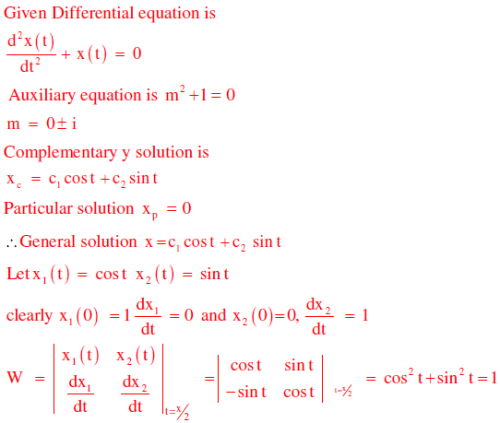Mechanical Engineering - ME 2014 GATE Paper (Practice Test) - Question 38

A machine produces 0, 1 or 2 defective pieces in a day with associated probability of 1/6, 2/3 and 1/6, respectively. The mean value and the variance of the number of defective pieces produced by the machine in a day, respectively, are

Detailed Solution for Mechanical Engineering - ME 2014 GATE Paper (Practice Test) - Question 38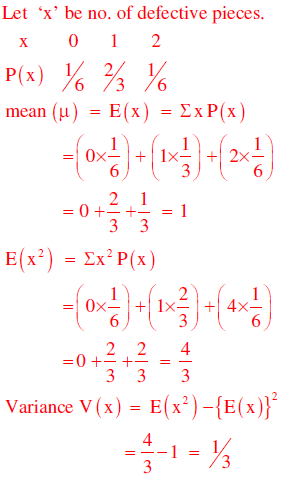*Answer can only contain numeric values
Mechanical Engineering - ME 2014 GATE Paper (Practice Test) - Question 39

The real root of the equation 5x − 2cosx −1 = 0 (up to two decimal accuracy) is _______

(Important - Enter only the numerical value in the answer)

Detailed Solution for Mechanical Engineering - ME 2014 GATE Paper (Practice Test) - Question 39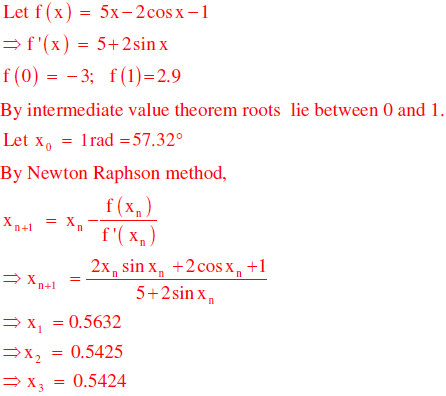*Answer can only contain numeric values
Mechanical Engineering - ME 2014 GATE Paper (Practice Test) - Question 40

A drum brake is shown in the figure. The drum is rotating in anticlockwise direction. The coefficient of friction between drum and shoe is 0.2. The dimensions shown in the figure are in mm. The braking torque (in N.m) for the brake shoe is _______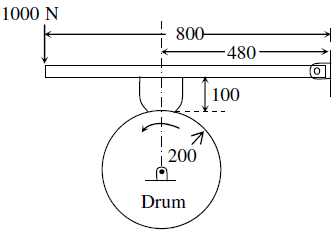(Important - Enter only the numerical value in the answer)

Detailed Solution for Mechanical Engineering - ME 2014 GATE Paper (Practice Test) - Question 40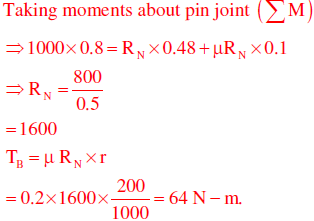*Answer can only contain numeric values
Mechanical Engineering - ME 2014 GATE Paper (Practice Test) - Question 41

A body of mass (M) 10 kg is initially stationary on a 45° inclined plane as shown in figure. The coefficient of dynamic friction between the body and the plane is 0.5. The body slides down the plane and attains a velocity of 20 m/s. The distance travelled (in meter) by the body along the plane is _______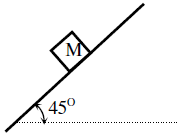(Important - Enter only the numerical value in the answer)

Detailed Solution for Mechanical Engineering - ME 2014 GATE Paper (Practice Test) - Question 41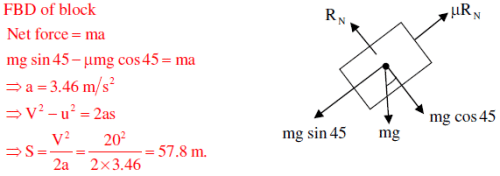Mechanical Engineering - ME 2014 GATE Paper (Practice Test) - Question 42

Consider a simply supported beam of length, 50h, with a rectangular cross-section of depth, h, and width, 2h. The beam carries a vertical point load, P, at its mid-point. The ratio of the maximum shear stress to the maximum bending stress in the beam is

Detailed Solution for Mechanical Engineering - ME 2014 GATE Paper (Practice Test) - Question 42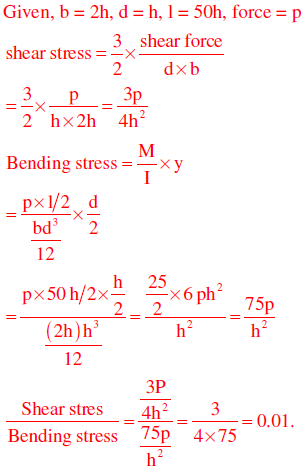*Answer can only contain numeric values
Mechanical Engineering - ME 2014 GATE Paper (Practice Test) - Question 43

The damping ratio of a single degree of freedom spring-mass-damper system with mass of 1 kg, stiffness 100 N/m and viscous damping coefficient of 25 N.s/m is _______

Detailed Solution for Mechanical Engineering - ME 2014 GATE Paper (Practice Test) - Question 43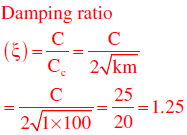Mechanical Engineering - ME 2014 GATE Paper (Practice Test) - Question 44

An annular disc has a mass m, inner radius R and outer radius 2R. The disc rolls on a flat surface without slipping. If the velocity of the centre of mass is v, the kinetic energy of the disc is

Detailed Solution for Mechanical Engineering - ME 2014 GATE Paper (Practice Test) - Question 44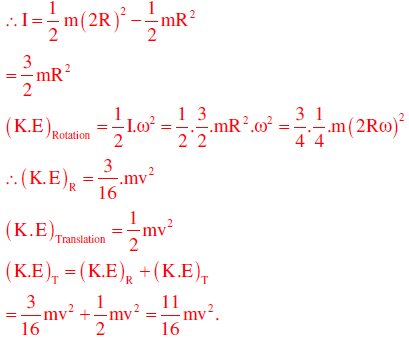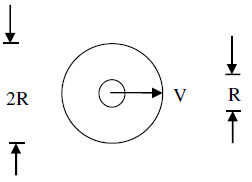Mechanical Engineering - ME 2014 GATE Paper (Practice Test) - Question 45

A force P is applied at a distance x from the end of the beam as shown in the figure. What would be the value of x so that the displacement at ‘A’ is equal to zero?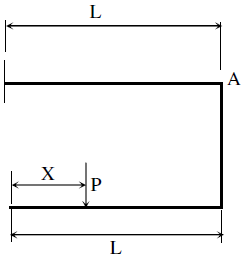Detailed Solution for Mechanical Engineering - ME 2014 GATE Paper (Practice Test) - Question 45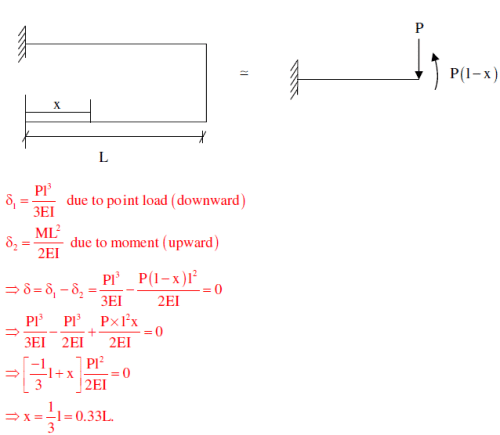Mechanical Engineering - ME 2014 GATE Paper (Practice Test) - Question 46

Consider a rotating disk cam and a translating roller follower with zero offset. Which one of the following pitch curves, parameterized by t, lying in the interval 0 to 2π, is associated with the maximum translation of the follower during one full rotation of the cam rotating about the center at (x, y) = (0, 0) ?

Detailed Solution for Mechanical Engineering - ME 2014 GATE Paper (Practice Test) - Question 46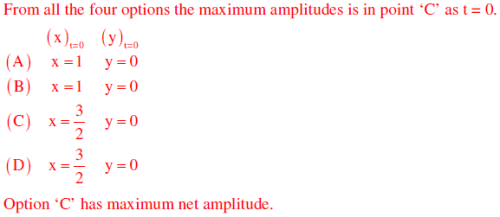*Answer can only contain numeric values
Mechanical Engineering - ME 2014 GATE Paper (Practice Test) - Question 47

A four-wheel vehicle of mass 1000 kg moves uniformly in a straight line with the wheels revolving at 10 rad/s. The wheels are identical, each with a radius of 0.2 m. Then a constant braking torque is applied to all the wheels and the vehicle experiences a uniform deceleration. For the vehicle to stop in 10 s, the braking torque (in N.m) on each wheel is _______

(Important - Enter only the numerical value in the answer)

Detailed Solution for Mechanical Engineering - ME 2014 GATE Paper (Practice Test) - Question 47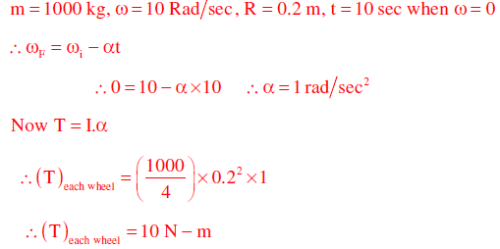*Answer can only contain numeric values
Mechanical Engineering - ME 2014 GATE Paper (Practice Test) - Question 48

A slider-crank mechanism with crank radius 60 mm and connecting rod length 240 mm is shown in figure. The crank is rotating with a uniform angular speed of 10 rad/s, counter clockwise. For the given configuration, the speed (in m/s) of the slider is _______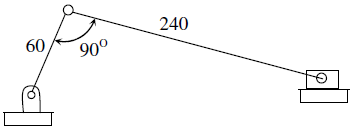(Important - Enter only the numerical value in the answer)

*Answer can only contain numeric values
Mechanical Engineering - ME 2014 GATE Paper (Practice Test) - Question 49

Consider an objective function Z(x1 ,x2) = 3x1 + 9x2 and the constraints
x1 + x2 < 8,

x1 + 2x2 < 4,

x1 >  x2 > 0,

The maximum value of the objective function is _______

Detailed Solution for Mechanical Engineering - ME 2014 GATE Paper (Practice Test) - Question 49

Roots are (12,-4) and (12,-2)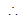Maximum value of objective function = 3(12)+9(-2) = 18.

*Answer can only contain numeric values
Mechanical Engineering - ME 2014 GATE Paper (Practice Test) - Question 50

A mass-spring-dashpot system with mass m = 10 kg, spring constant k = 6250 N/m is excited by a harmonic excitation of 10 cos(25t) N. At the steady state, the vibration amplitude of the mass is 40 mm. The damping coefficient (c, in N.s/m) of the dashpot is _______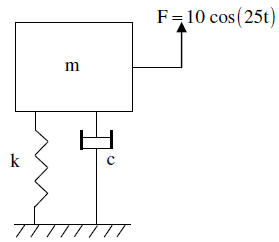Detailed Solution for Mechanical Engineering - ME 2014 GATE Paper (Practice Test) - Question 50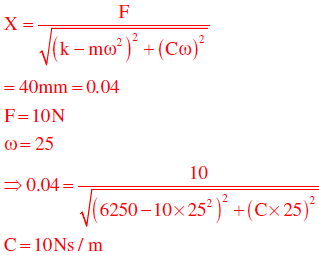Mechanical Engineering - ME 2014 GATE Paper (Practice Test) - Question 51

A certain amount of an ideal gas is initially at a pressure P1 and temperature T1. First, it undergoes a constant pressure process 1-2 such that T2 = 3T1/4. Then, it undergoes a constant volume process 2-3 such that T3 = T1/2. The ratio of the final volume to the initial volume of the ideal gas is

Detailed Solution for Mechanical Engineering - ME 2014 GATE Paper (Practice Test) - Question 51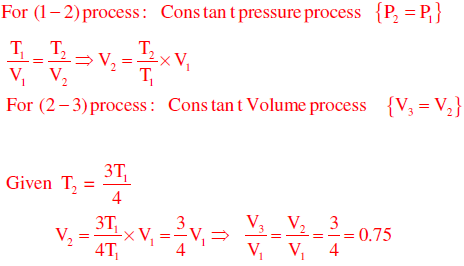*Answer can only contain numeric values
Mechanical Engineering - ME 2014 GATE Paper (Practice Test) - Question 52

An amount of 100 kW of heat is transferred through a wall in steady state. One side of the wall is maintained at 127OC and the other side at 27OC. The entropy generated (in W/K) due to the heat transfer through the wall is _______

(Important - Enter only the numerical value in the answer)

Detailed Solution for Mechanical Engineering - ME 2014 GATE Paper (Practice Test) - Question 52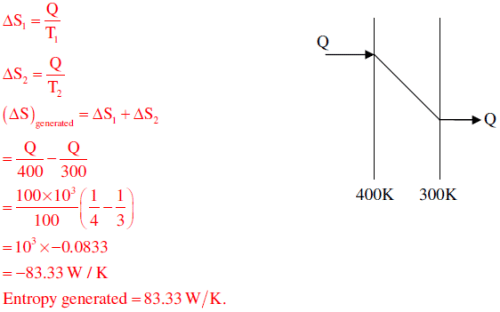Mechanical Engineering - ME 2014 GATE Paper (Practice Test) - Question 53

A siphon is used to drain water from a large tank as shown in the figure below. Assume that the level of water is maintained constant. Ignore frictional effect due to viscosity and losses at entry and exit. At the exit of the siphon, the velocity of water is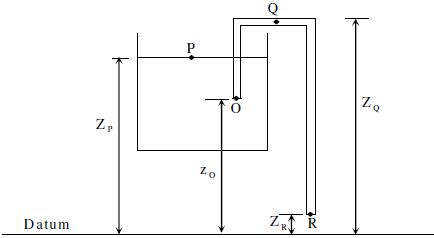Detailed Solution for Mechanical Engineering - ME 2014 GATE Paper (Practice Test) - Question 53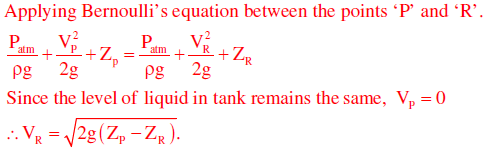*Answer can only contain numeric values
Mechanical Engineering - ME 2014 GATE Paper (Practice Test) - Question 54

Heat transfer through a composite wall is shown in figure. Both the sections of the wall have equal thickness (l). The conductivity of one section is k and that of the other is 2k. The left face of the wall is at 600 K and the right face is at 300 K. The interface temperature Ti (in K) of the composite wall is _______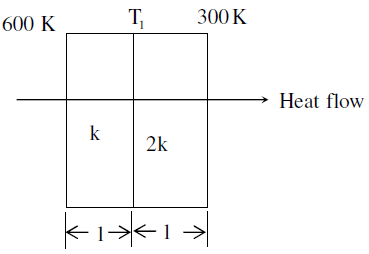Detailed Solution for Mechanical Engineering - ME 2014 GATE Paper (Practice Test) - Question 54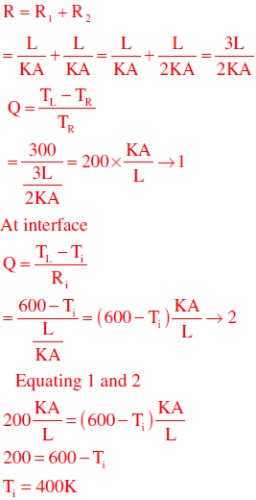*Answer can only contain numeric values
Mechanical Engineering - ME 2014 GATE Paper (Practice Test) - Question 55

A fluid of dynamic viscosity 2 × 10-5 kg/m.s and density 1 kg/m3 flows with an average velocity of 1 m/s through a long duct of rectangular (25 mm × 15 mm) cross-section. Assuming laminar flow, the pressure drop (in Pa) in the fully developed region per meter length of the duct is _______

(Important - Enter only the numerical value in the answer)

Detailed Solution for Mechanical Engineering - ME 2014 GATE Paper (Practice Test) - Question 55

Given,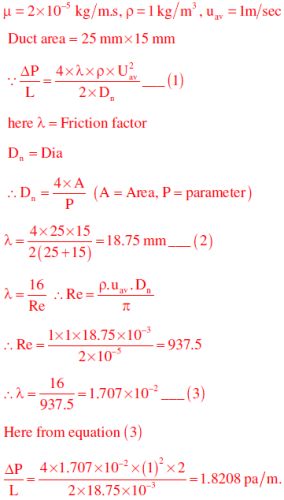*Answer can only contain numeric values
Mechanical Engineering - ME 2014 GATE Paper (Practice Test) - Question 56

At the inlet of an axial impulse turbine rotor, the blade linear speed is 25 m/s, the magnitude of absolute velocity is 100 m/s and the angle between them is 25°. The relative velocity and the axial component of velocity remain the same between the inlet and outlet of the blades. The blade inlet and outlet velocity triangles are shown in the figure. Assuming no losses, the specific work (in J/kg) is _______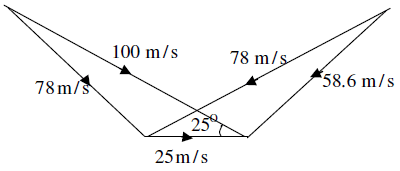(Important - Enter only the numerical value in the answer)

Detailed Solution for Mechanical Engineering - ME 2014 GATE Paper (Practice Test) - Question 56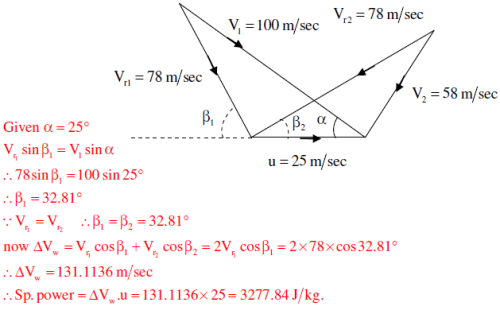Mechanical Engineering - ME 2014 GATE Paper (Practice Test) - Question 57

A solid sphere of radius r1 = 20 mm is placed concentrically inside a hollow sphere of radius r2 = 30 mm as shown in the figure.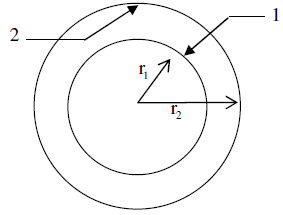The view factor F21 for radiation heat transfer is

Detailed Solution for Mechanical Engineering - ME 2014 GATE Paper (Practice Test) - Question 57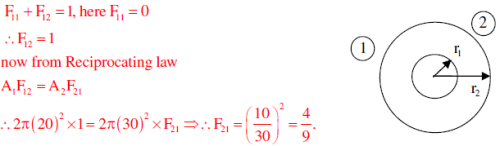*Answer can only contain numeric values
Mechanical Engineering - ME 2014 GATE Paper (Practice Test) - Question 58

A double-pipe counter-flow heat exchanger transfers heat between two water streams. Tube side water at 19 liter/s is heated from 10OC to 38OC. Shell side water at 25 liter/s is entering at 46OC. Assume constant properties of water, density is 1000 kg/m3 and specific heat is 4186 J/kg K. The LMTD (in OC) is ________

(Important - Enter only the numerical value in the answer)

Detailed Solution for Mechanical Engineering - ME 2014 GATE Paper (Practice Test) - Question 58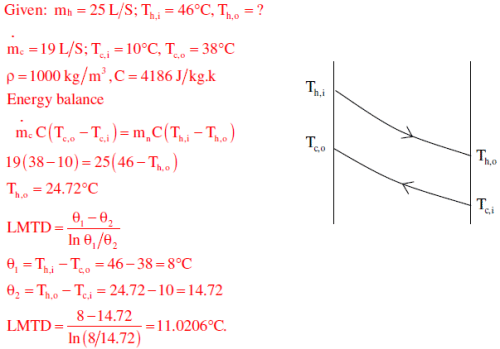*Answer can only contain numeric values
Mechanical Engineering - ME 2014 GATE Paper (Practice Test) - Question 59

A diesel engine has a compression ratio of 17 and cut-off take place at 10% of the stroke. Assuming ratio of specific heats (g ) as 1.4, the air-standard efficiency (in percent) is_______

(Important - Enter only the numerical value in the answer)

Detailed Solution for Mechanical Engineering - ME 2014 GATE Paper (Practice Test) - Question 59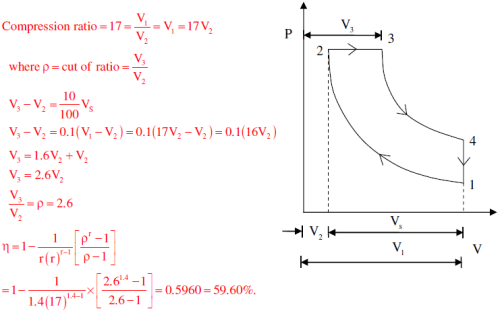Mechanical Engineering - ME 2014 GATE Paper (Practice Test) - Question 60

Consider the given project network, where numbers along various activities represent the normal time. The free float on activity 4-6 and the project duration, respectively, are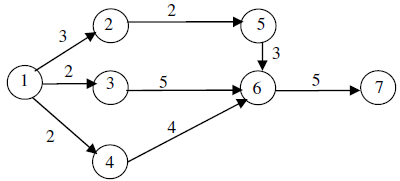Detailed Solution for Mechanical Engineering - ME 2014 GATE Paper (Practice Test) - Question 60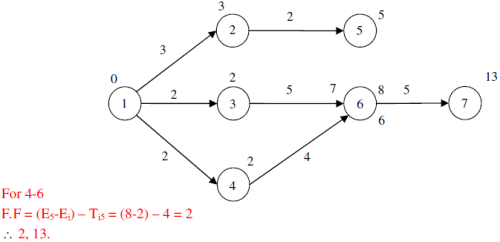Mechanical Engineering - ME 2014 GATE Paper (Practice Test) - Question 61

A manufacturer can produce 12000 bearings per day. The manufacturer received an order of 8000 bearings per day from a customer. The cost of holding a bearing in stock is Rs.0.20 per month. Setup cost per production run is Rs.500. Assuming 300 working days in a year, the frequency of production run should be

Mechanical Engineering - ME 2014 GATE Paper (Practice Test) - Question 62

A cylindrical blind riser with diameter d and height h, is placed on the top of the mold cavity of a closed type sand mold as shown in the figure. If the riser is of constant volume, then the rate of solidification in the riser is the least when the ratio h/d is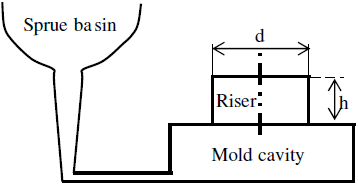Detailed Solution for Mechanical Engineering - ME 2014 GATE Paper (Practice Test) - Question 62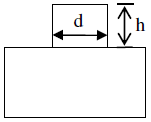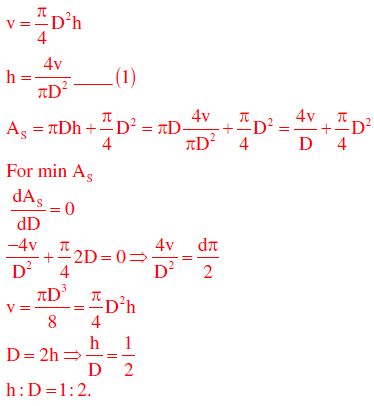*Answer can only contain numeric values
Mechanical Engineering - ME 2014 GATE Paper (Practice Test) - Question 63

The diameter of a recessed ring was measured by using two spherical balls of diameter d2 =60 mm and d= 40 mm as shown in the figure.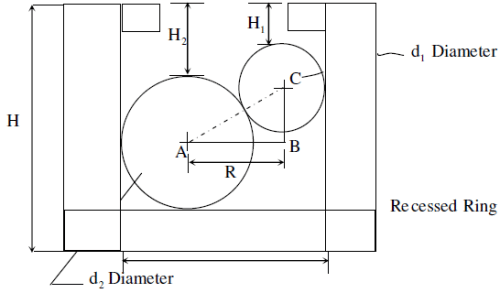The distance H2 = 35.55 mm and H1 = 20.55 mm. The diameter (D, in mm) of the ring gauge is _______

Detailed Solution for Mechanical Engineering - ME 2014 GATE Paper (Practice Test) - Question 63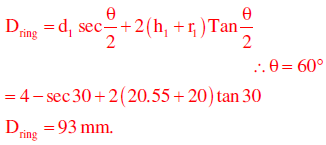Mechanical Engineering - ME 2014 GATE Paper (Practice Test) - Question 64

Which pair of following statements is correct for orthogonal cutting using a single-point cutting tool?

P. Reduction in friction angle increases cutting force
Q. Reduction in friction angle decreases cutting force
R. Reduction in friction angle increases chip thickness
S. Reduction in friction angle decreases chip thickness

*Answer can only contain numeric values
Mechanical Engineering - ME 2014 GATE Paper (Practice Test) - Question 65

For spot welding of two steel sheets (base metal) each of 3 mm thickness, welding current of 10000 A is applied for 0.2s. The heat dissipated to the base metal is 1000 J. Assuming that the heat required for melting 1 mm3 volume of steel is 20 J and interfacial contact resistance between sheets is 0.000 2? , the volume (in mm3) of weld nugget is _______

(Important - Enter only the numerical value in the answer)

Detailed Solution for Mechanical Engineering - ME 2014 GATE Paper (Practice Test) - Question 65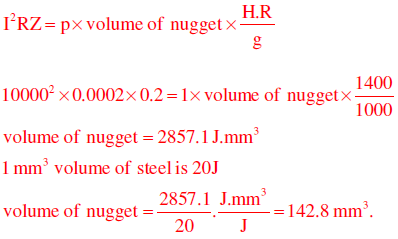## GATE Mechanical (ME) 2023 Mock Test Series

27 docs|243 tests
Information about Mechanical Engineering - ME 2014 GATE Paper (Practice Test) Page
In this test you can find the Exam questions for Mechanical Engineering - ME 2014 GATE Paper (Practice Test) solved & explained in the simplest way possible. Besides giving Questions and answers for Mechanical Engineering - ME 2014 GATE Paper (Practice Test), EduRev gives you an ample number of Online tests for practice

## GATE Mechanical (ME) 2023 Mock Test Series

27 docs|243 tests(Scan QR code)